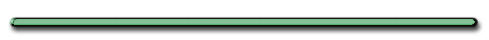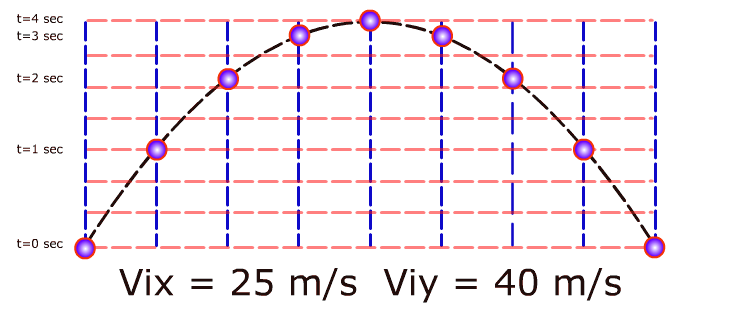Projectile Motion
Show Me The Physics WebsiteProjectile

Where Vix = 25 m/s

Viy = 40 m/s

 t (sec) Vx (m/s) Vy (m/s) 0 25 40 1 25 30 2 25 20 3 25 10 4 25 0 (peak) 5 25 -10 6 25 -20 7 25 -30 8 25 -40

A projectile is fired at an angle q and a velocity V. The object returns to level ground.

Express the time it takes for the object to hit the ground in terms of V, q and any other relevant variables

Viy = Visinq

Vfy = -Visinq

Vfy = Viy + at

-Visinq = Visinq - gt

Total time - Level Ground

t = (2Visin
q)/g

4. Word Problems

www.sihunt.co.uk Russian long jumper Tayana Lebedeva performs a
double hitch action in the 2007 world finals in Osaka Japan.

 Ex) An athlete doing a running jump leaves ground at an angle of 25.° and a velocity of 10. m/s. (a) How long does it take athlete to reach her maximum height?Viy = VisinӨ

= (10. m/s)sin25.

= 4.2 m/s up

 X Y t = ? Viy = 4.2 m/s (from before) t  =  ? Vfy= 0 (peak) ay = -9.8 m/s2

Vf = Vi + at

0 =4.2 m/s+(-9.8 m/s2)t

(subtract 4.2 m/s from both sides)

- 4.2 m/s = (-10. m/s2)t

(- 4.2 m/s)/(-10. m/s2) = t

t = .42 secs
to reach peak

(b) How far did she jump?

On level ground

 Total time = double peak time

Entire Jump:

Total Time = .84 seconds

dx = ?

dx = ?

t = .86 sec

dx = Vxt

(Must find Vx first)

Vx = VcosӨ

Vx = (10. m/s)cos25.

Vx = 9.1 m/s

dx = 9.1 m/s(.84 sec)

dx = 7.6 m[AP]